Next: Collisional Conservation Laws Up: Collisions Previous: Two-Body Elastic Collisions

# Boltzmann Collision Operator

Let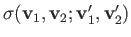be the cross-section for a scattering process by which particles of types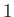and(located at position vector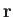at time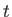) are incident with velocities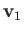and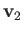, respectively, and are scattered to velocities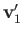and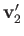, respectively (Reif 1965). Assuming that the scattering process is reversible in time and space (which is certainly the case for two-body Coulomb collisions), the cross-section for the inverse process must be the same as that for the forward process (Reif 1965). In other words,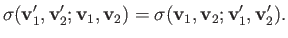(3.16)

The rate at which particles with the original velocitiesandare scattered into the rangeto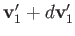and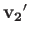to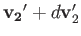is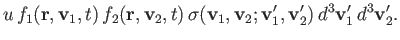(3.17)

Here,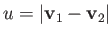. Moreover,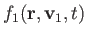and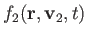are the ensemble-averaged distribution functions for particles of typesand, respectively. In writing the previous expression, we have assumed that the distribution functions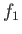and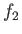are uncorrelated. This assumption is reasonable provided that the mean-free-path is much longer than the effective range of the inter-particle force. (This follows because, before they encounter one another, two colliding particles originate at different points that are typically separated by a mean-free-path. However, the typical correlation length is of similar magnitude to the range of the inter-particle force.) In writing the previous expression, we have also implicitly assumed that the inter-particle force responsible for the collisions is sufficiently short-range that the particle position vectors do not change appreciably (on a macroscopic lengthscale) during a collision. (Both of the previous assumptions are valid in a conventional weakly coupled plasma, because the range of the inter-particle force is of order the Debye length, which is assumed to be much smaller than any macroscopic lengthscale. Moreover, the mean-free-path is much longer than the Debye length--see Section 1.7.) By analogy with Equation (3.17), the rate at which particles with the original velocitiesandare scattered into the rangeto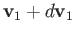and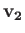to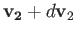is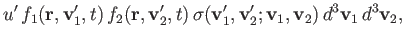(3.18)

where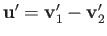. Now, it is easily demonstrated from Equations (3.12) and (3.13) that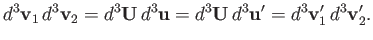(3.19)

The result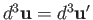follows from the fact that the vectors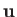and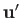differ only in direction. Thus, the net rate of change of the distribution function of particles of typewith velocities(at positionand time) due to collisions with particles of type[i.e., the collision operator--see Equation (3.9)] is given by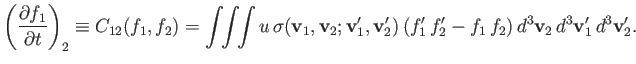(3.20)

Here, use has been made of Equation (3.16), as well as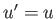. Moreover,,.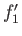, and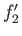are short-hand for,,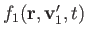, and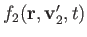, respectively. The previous expression is known as the Boltzmann collision operator. By an analogous argument, the net rate of change of the distribution function of particles of typewith velocities(at positionand time) due to collisions with particles of typeis given by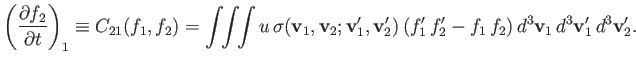(3.21)

Expression (3.20) for the Boltzmann collision operator can be further simplified for elastic collisions because, in this case, the collision cross-sectionis a function only of the magnitude of the relative velocity vector,, and its change in direction as a result of the collision. Furthermore, the integral over the final velocitiesandreduces to an integral over all solid angles for the change in direction of. Thus, we can write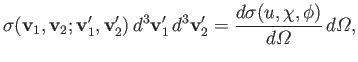(3.22)

where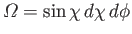. Here,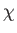is the angle through which the direction ofis deflected as a consequence of the collision (see Figure 3.1), and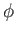is an azimuthal angle that determines the orientation of the plane in which the vectoris confined during the collision. (See Section 3.7.) Moreover,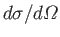is a conventional differential scattering cross-section (Reif 1965). Hence, we obtain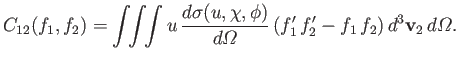(3.23)

Note, finally, that if we exchange the identities of particlesandin Equation (3.22) then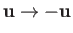, but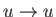,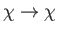, and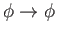. Thus, we conclude that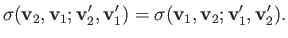(3.24)Next: Collisional Conservation Laws Up: Collisions Previous: Two-Body Elastic Collisions
Richard Fitzpatrick 2016-01-23